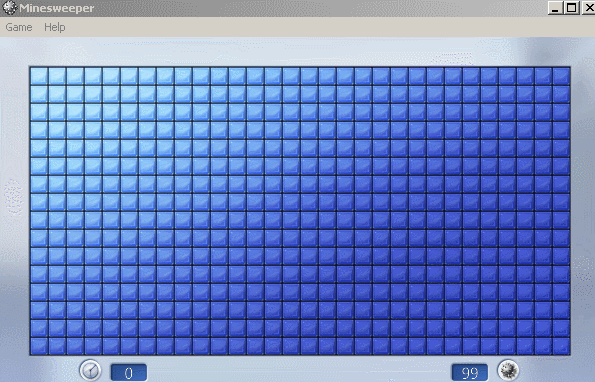Minesweeper is a single-player computer game where an N x N square matrix has certain number of mines, i.e, bombs, hidden across the board. The remaining cells are either blank or have a number behind them. The numbers represent the number of bombs hidden in the surrounding eight cells. If the cell is blank, all adjacent blank cells (up to and including the surrounding numeric cells) would be exposed.

The player wins when all non-bomb cells are exposed. The game also allows the player to flag certain cells as potential bombs. Flagging does not affect the game other than just to block the cells from accidentally getting clicked.

Below is This is a fully exposed board with 3 bombs. This is not shown to the user. The objective of the player is to discover this.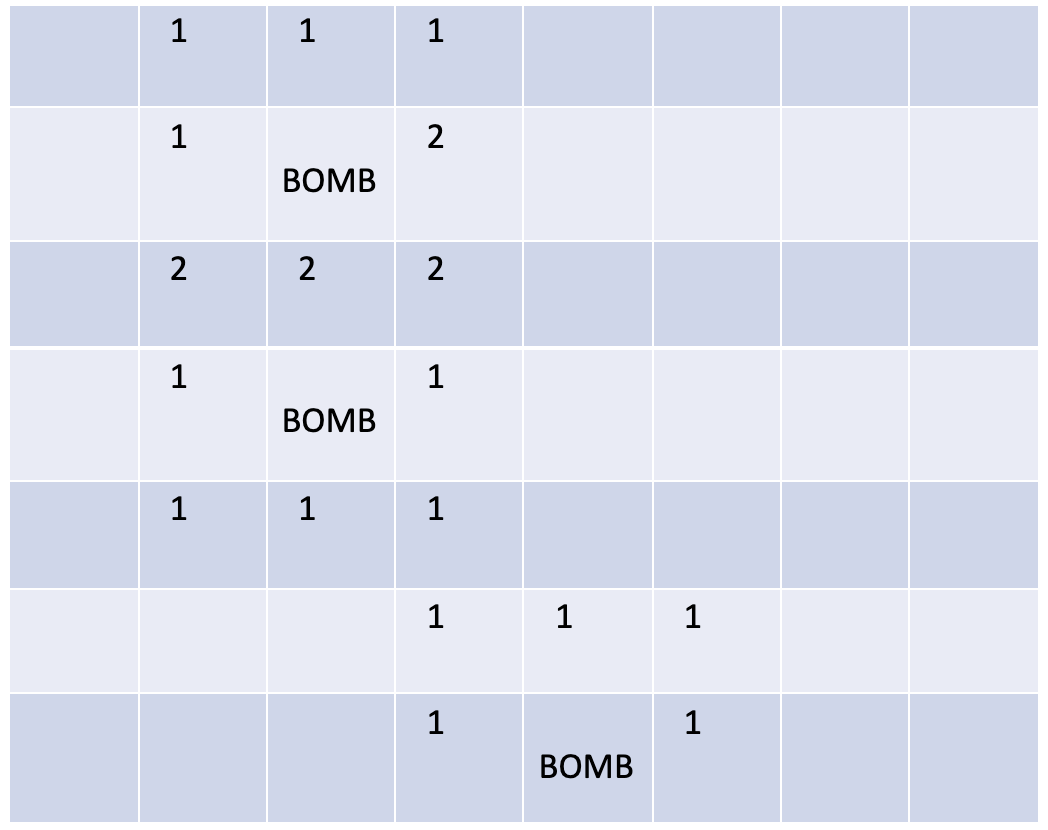The player initially sees a board with nothing exposed like below.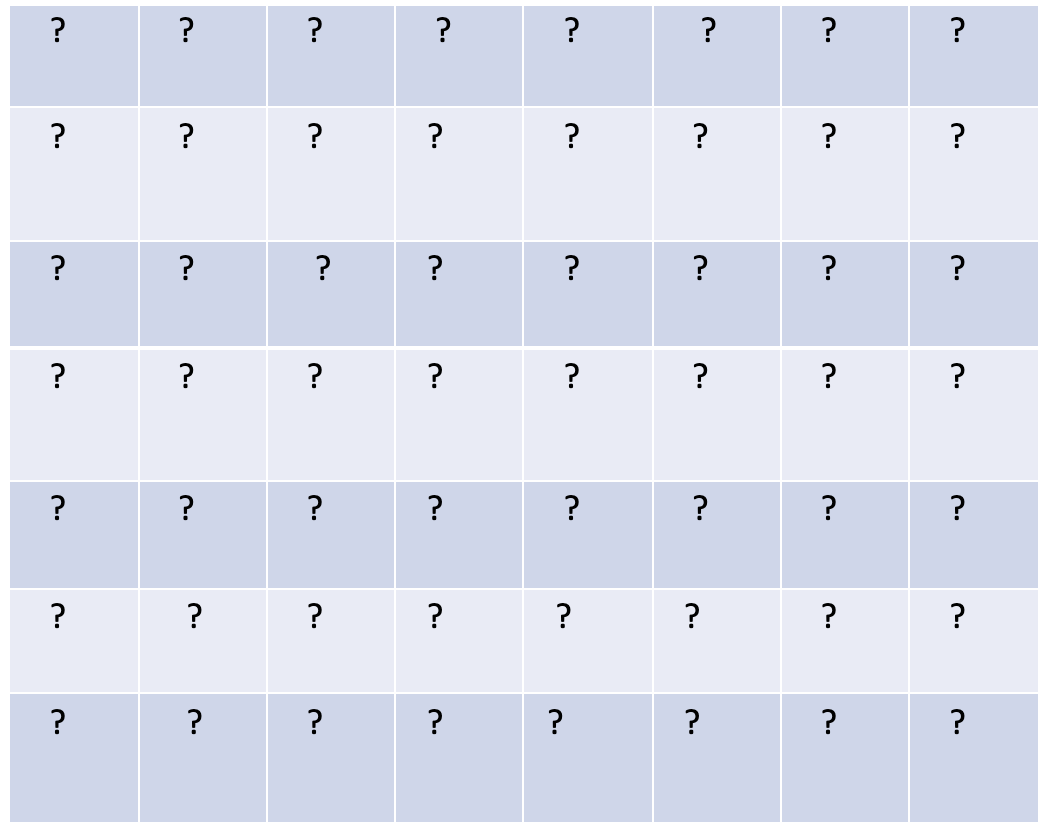Clicking on cell (row= 1, col= 0) would expose this: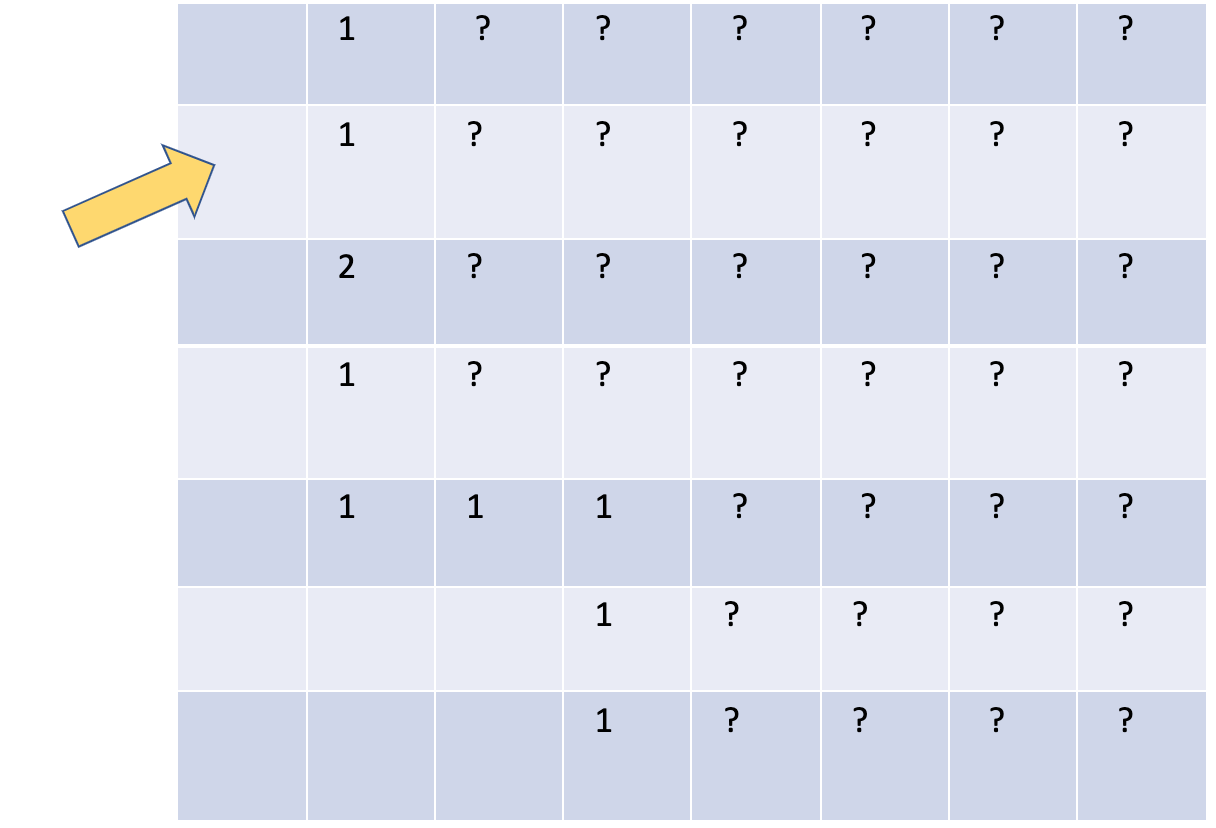The user wins when everything other than bombs has been exposed.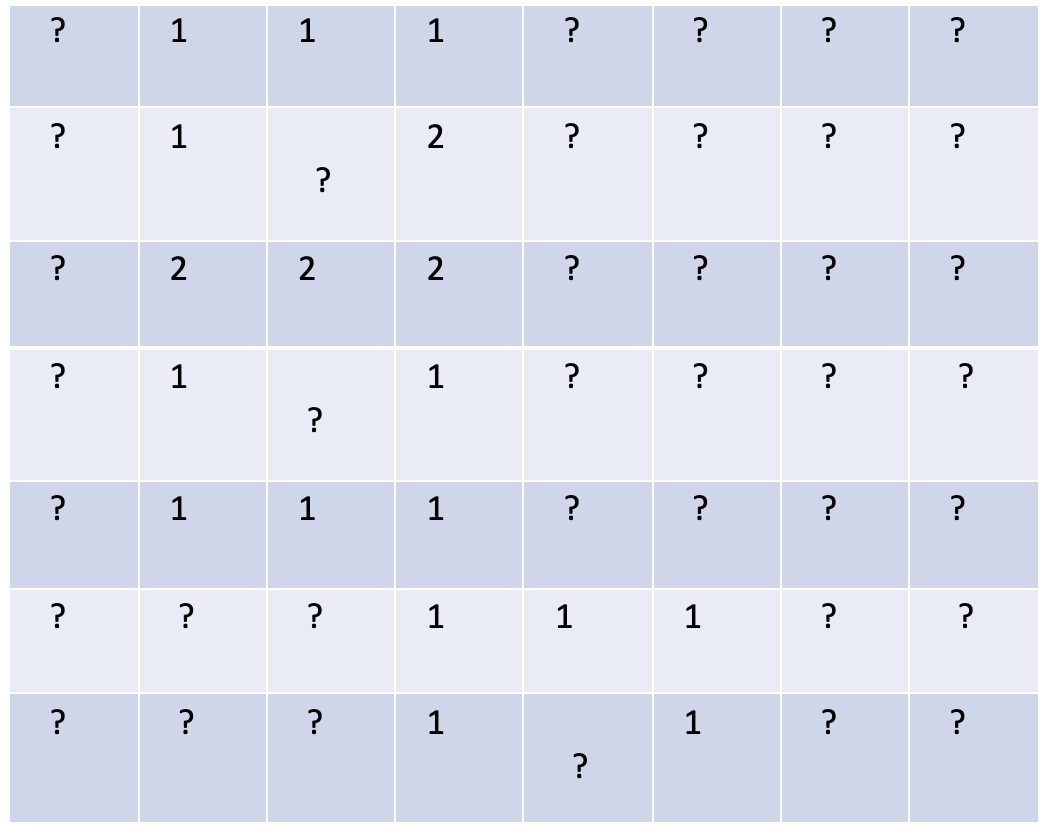How would you go about designing the game ?

#### Object Oriented Design:

The board represents the Minesweeper game and the board consists of Cell. So at the very least we would need Board and Cell classes. Now we would also need a Controller that would actually construct the board when a new instance of the game is created, keeps track of state of the game throughout the lofecycle of the game, as well as handle user input. So below are the classes we would need to design:
• Cell
• Board
• Game (This is the controller class.)
We will now dive deeper into each of the class design.

#### Cell

Let's jot down all the information we have about the minesweeper board cells:
• A Cell should always have an assigned coordinate i.e, row index and column index in the board.

• A cell can contain either a Bomb, or a Number, or could be Blank.

We can design for this in two ways:
1. We could have an enumerator as follows:
``````
public enum TYPE {
BOMB,
NUMBER,
BLANK
}``````

2. We can represent what kind of a cell one is just by using integers:
• If a cell is a Number then the number would be greater than equal to one. So if value of a cell is greater than zero we would know the cell is of type Number.
• We can represent a Blank cell using the interger value 0.
• We can use -1 to represent a cell with Bomb.
In an interview, you should speak out loud all the alternative ways you can achieve something. Just make sure you are not spending too much time doing that.

• A Cell could be in either of these two states:
• Exposed
• Unexposed
From our discussion above we get the below class implementation:

#### Java:

``````
package OOD.Minesweeper;

/**
* Created by Abhishek on 9/14/21.
*/
public class Cell {
private int row;
private int column;
private boolean isBomb;
private int number;
private boolean isExposed;
private boolean isFlagged;

public Cell(int r, int c) {
row = r;
column = c;
}

public void setRowAndColumn(int r, int c) {
row = r;
column = c;
}

public void setBomb() {
isBomb = true;
number = -1;
}

public void flag() {
isFlagged = true;
}

public void unflag() {
isFlagged = false;
}

public boolean isBlank() {
return number == 0;
}

public boolean isNumber() {
return number > 0;
}

public boolean isExposed() {
return isExposed;
}

public void expose() {
isExposed = true;
}

public int getNumber() {
return number;
}

public void setNumber(int number) {
this.number = number;
}

public int getRow() {
return row;
}

public int getColumn() {
return column;
}

public boolean isBomb() {
return isBomb;
}
}
```

```

#### Python:

``````
class Cell:
def __init__(self, r, c):
self._isBomb = False
self._number = 0
self._isExposed = False
self._isFlagged = False

self._row = r
self._column = c

def setRowAndColumn(self, r, c):
self._row = r
self._column = c

def setBomb(self):
self._isBomb = True
self._number = -1

def flag(self):
self._isFlagged = True

def unflag(self):
self._isFlagged = False

def isBlank(self):
return self._number == 0

def isNumber(self):
return self._number > 0

def isExposed(self):
return self._isExposed

def expose(self):
self._isExposed = True

def getNumber(self):
return self._number

def setNumber(self, number):
self._number = number

def getRow(self):
return self._row

def getColumn(self):
return self._column

def isBomb(self):
return self._isBomb

``````

#### Board

Board is a 2 dimensional square matrix of Cell objects. Board has the below reponsibilities:
• Being able to initialize the board and randomly place the blank cells, numbered cells and bombs.
• Being able to expose a cell when the player clicks on the cell.
• Being able to flag a cell as per player's instruction.
• Being able to keep of track of number of unexposed non-bomb cells at all time. This is important to determine when a player wins. A player wins when this number drops to zero.
• Being able to carry out below operation:
If player clicks on a blank cell, all adjacent blank cells (up to and including the surrounding numeric cells) would be exposed
Based on the above discussion we come up with below class implementation:

#### Java:

``````
package OOD.Minesweeper;

import java.util.Queue;
import java.util.Random;

/**
* Created by Abhishek on 9/15/21.
*/
public class Board {
public enum GameState { INPROGRESS, WON, LOST}
private int rows;
private int columns;
private Cell[][] cells;
private int unexposedCellsRemaining; // determines if a player wins

public Board(int rows, int columns, int numberOfBombsToBePlanted) {
this.rows = rows;
this.columns = columns;

Cell[] bombs = new Cell[numberOfBombsToBePlanted];

initializeBoard();
plantBombsAtRandomPositions(numberOfBombsToBePlanted, bombs);
setNumberedCells(bombs);

unexposedCellsRemaining = rows * columns - numberOfBombsToBePlanted;
}

private void initializeBoard() {
cells = new Cell[rows][columns];
for (int r = 0; r < rows; r++) {
for (int c = 0; c < columns; c++) {
cells[r][c] = new Cell(r, c);
}
}
}

private void plantBombsAtRandomPositions(int numberOfBombsToBePlanted, Cell[] bombs) {
// place bombs randomly across the board
}

private void setNumberedCells(Cell[] bombs) {
// set all the numbered cells depending on how the bombs are placed on the board

}

// makes a move as per player's instruction
// and returns the state of the game: game lost, or, game still in progress, or, player won
public GameState exposeCell(int row, int col) {
Cell cell = cells[row][col];
if (!cell.isExposed()) {
cell.expose();
if (cell.isBlank()) {
expandBlank();
}
unexposedCellsRemaining--;
if (unexposedCellsRemaining == 0) {
return GameState.WON;
}
if (cell.isBomb()) {
return GameState.LOST;
}
}
return GameState.INPROGRESS;
}

private void expandBlank(Cell cell) {
// expose all adjacent blank cells, up to
// and including the surrounding numeric cells
}

public void printBoard() {
// print the board
}

}

```

```

#### Python:

``````
from enum import Enum

from OOD.Minesweeper.Cell import Cell

class GameState(Enum):
INPROGRESS = 0
WON = 1
LOST = 2

class Board:
def __init__(self, rows, columns, numberOfBombsToBePlanted):
self._cells = [[]]
self._unexposedCellsRemaining = 0

self._rows = rows
self._columns = columns
bombs = [None for _ in range(numberOfBombsToBePlanted)]
self._initializeBoard()
self._plantBombsAtRandomPositions(numberOfBombsToBePlanted, bombs)
self._setNumberedCells(bombs)
self._unexposedCellsRemaining = rows * columns - numberOfBombsToBePlanted

def _initializeBoard(self):
self._cells = [[] for _ in range(self._rows)]
r = 0
while r < self._rows:
c = 0
while c < self._columns:
self._cells[r][c] = Cell(r, c)
c += 1
r += 1

def _plantBombsAtRandomPositions(self, numberOfBombsToBePlanted, bombs):
pass

def _setNumberedCells(self, bombs):

pass

def exposeCell(self, row, col):
cell = self._cells[row][col]
if not cell.isExposed():
cell.expose()
if cell.isBlank():
self._expandBlank()
self._unexposedCellsRemaining -= 1
if self._unexposedCellsRemaining == 0:
return GameState.WON
if cell.isBomb():
return GameState.LOST
return GameState.INPROGRESS

def _expandBlank(self, cell):

pass

def printBoard(self):

pass

``````

#### Game

The Game class takes instructions from the user (i.e, player) and carries them out. Game class should have the capability to initialize a board, start the game and determine when a player wins or loses.

#### Java:

``````
package OOD.Minesweeper;

import java.util.Scanner;

/**
* Created by Abhishek on 9/15/21.
*/
public class Game {

private Board board;

private int boardRows;
private int boardColumns;
private int numberOfBombsInTheGame;

public Game(int boardRows, int boardColumns, int numberOfBombs) {
this.boardRows = boardRows;
this.boardColumns =  boardColumns;
this.numberOfBombsInTheGame = numberOfBombs;
}

private void initialize() {
if (board == null) {
board = new Board(boardRows, boardColumns, numberOfBombsInTheGame);
board.printBoard(); // display board
}
}

public void start() {
if (board == null) {
initialize();
}
playGame();
}

private void playGame() {
Scanner scanner = new Scanner(System.in);
Board.GameState gameState = Board.GameState.INPROGRESS;

while (gameState == Board.GameState.INPROGRESS) {
String input = scanner.nextLine(); // input the coordinate of the cell you want to click in the form of row-col
if (input.equals("exit")) { // exit is the keyword to exit the game
scanner.close();
return;
}

int row = Integer.parseInt(input.split("-"));
int col = Integer.parseInt(input.split("-"));

gameState = board.exposeCell(row, col);

// check if the player won or lost by making the above move
if (gameState == Board.GameState.LOST) {
System.out.println("FAIL");
} else if (gameState == Board.GameState.WON) {
System.out.println("WIN");
}

board.printBoard(); // display board after the player has made the move
}
scanner.close();
}

}
```
```

#### Python:

``````
from OOD.Minesweeper.Board import Board, GameState

class Game:
def __init__(self, boardRows, boardColumns, numberOfBombs):
self._board = None
self._boardRows = boardRows
self._boardColumns = boardColumns
self._numberOfBombsInTheGame = numberOfBombs
def _initialize(self):
if self._board is None:
self._board = Board(self._boardRows, self._boardColumns, self._numberOfBombsInTheGame)
self._board.printBoard()
def start(self):
if self._board is None:
self._initialize()
self._playGame()
def _playGame(self):
scanner = input()
gameState = GameState.INPROGRESS
while gameState is GameState.INPROGRESS:
inputi = input()
if inputi == "exit":
return
row = int(inputi.split("-"))
col = int(inputi.split("-"))
gameState = self._board.exposeCell(row, col)
if gameState is GameState.LOST:
print("FAIL")
elif gameState is GameState.WON:
print("WIN")
self._board.printBoard()
``````

#### Low Level Implementation:

```    This is where your interviewer would ask you to
go deeper in some of the features and do a low level implementation.
We would focus on desiging the algorithms and coming up with low level design of

expandBlank(cell),

setNumberedCells(Cell[] bombs),

shuffleBombs().

Placing the bombs randomly across the board:
We randomize the positions of bombs across the board by using a very sophisticated
shuffle algorithm called
Fisher-Yates Shuffling
which is discussedin our Algorithms course.

We
would place the K bombs in the first K cells and then shuffle all the cells around.

Java:

```

private void plantBombsRandomly(int numberOfBombsToBePlanted, Cell[] bombs) {
plantBombsSequentially(numberOfBombsToBePlanted, bombs);
shuffleBombs();
}
private void plantBombsSequentially(int numberOfBombs, Cell[] bombs) {
for (int i = 0; i < numberOfBombs; i++) {
int r = i / columns;
int c = (i - r * columns) % columns;
cells[r][c].setBomb();
bombs[i] = cells[r][c];
}
}

private void shuffleBombs() {
int nCells = rows * columns;
Random random = new Random();

for (int index1 = 0; index1 < nCells; index1++) {

int index2 = index1 + random.nextInt(nCells - index1);

if (index1 != index2) {

// compute row and column index from index1 value
int row1 = index1 / columns;
int column1 = (index1 - row1 * columns) % columns;

Cell cell1 = cells[row1][column1];

// compute row and column index corresponsing to index2
int row2 = index2 / columns;
int column2 = (index2 - row2 * columns) % columns;

Cell cell2 = cells[row2][column2];

// swap
cells[row1][column1] = cell2;
cell2.setRowAndColumn(row1, column1);
cells[row2][column2] = cell1;
cell1.setRowAndColumn(row2, column2);
}
}
}

```

Python:

```

def _plantBombsRandomly(self, numberOfBombsToBePlanted, bombs):
self._plantBombsSequentially(numberOfBombsToBePlanted, bombs)
self._shuffleBombs()

def _shuffleBombs(self):
nCells = self._rows * self._columns
for index1 in range(0, nCells):
index2 = index1 + random.randint(nCells - index1)
if index1 != index2:

row1 = index1 / self._columns
column1 = int(math.fmod((index1 - row1 * self._columns), self._columns))
cell1 = self._cells[row1][column1]

row2 = index2 / self._columns
column2 = int(math.fmod((index2 - row2 * self._columns), self._columns))
cell2 = self._cells[row2][column2]
self._cells[row1][column1] = cell2
cell2.setRowAndColumn(row1, column1)
self._cells[row2][column2] = cell1
cell1.setRowAndColumn(row2, column2)

def _plantBombsSequentially(self, numberOfBombs, bombs):
for i in range(0, numberOfBombs):

r = i / self._columns
c = int(math.fmod((i - r * self._columns), self._columns))
self._cells[r][c].setBomb()
bombs[i] = self._cells[r][c]

```

Set the numbered cells:
Now that all the bombs are planted in random cells
throughout the board, we go to each bomb and update the surrounding
non-bomb cells with appropriate number.

Java:

```
private void setNumberedCells(Cell[] bombs) {
int[][] directions = { // Offsets of 8 surrounding cells
{-1, -1}, {-1, 0}, {-1, 1},
{ 0, -1},          { 0, 1},
{ 1, -1}, { 1, 0}, { 1, 1}
};
for (Cell bomb : bombs) {
int row = bomb.getRow();
int col = bomb.getColumn();
for (int[] direction : directions) {
int r = row + direction;
int c = col + direction;
if (r >= 0 && r < rows && c >= 0 && c < columns) {
cells[r][c].setNumber(cells[r][c].getNumber() + 1);
}
}
}
}
```

Python:

```
def _setNumberedCells(self, bombs):
directions = [[-1, -1], [-1, 0], [-1, 1], [0, -1], [0, 1], [1, -1], [1, 0], [1, 1]]
for bomb in bombs:
row = bomb.getRow()
col = bomb.getColumn()
for direction in directions:
r = row + direction
c = col + direction
if 0 <= r < self._rows and 0 <= c < self._columns:
self._cells[r][c].setNumber(self._cells[r][c].getNumber() + 1)

```

Expand a Blank Region:
If you think profoundly, you would figire out that
each blank cell is surrounded either by blank cells or numbered
cells, and never a bomb. The fact that there is no bomb cell surounding a blank cell is the reason
why a blank cell never gets number in the first place. A blank cell can be imagined as a cell with number value = 0, which means
0 bombs surrounding it.

All surrounding cells, blank and numbered, need to be exposed.
But, if you're exposing a blank cell, you also need to add the blank
cells to a queue of cells that need to be further expanded. We do not expand on a number cell, we only
expose them. But for a neighbor cell
which is a blank
cell, we expose them and then keep expanding on them.

We would implement our search algorithm using

Breadth First Search, which is discussed in-depth
in our Algorithms course.

Java:

```
public void expandBlank(Cell cell) {
int[][] directions = {
{-1, -1}, {-1, 0}, {-1, 1},
{ 0, -1},          { 0, 1},
{ 1, -1}, { 1, 0}, { 1, 1}
};

while (!toExplore.isEmpty()) {
Cell current = toExplore.remove();

for (int[] direction : directions) {
int r = current.getRow() + direction;
int c = current.getColumn() + direction;

if (r >= 0 && r < rows && c >= 0 && c < columns) {
Cell neighbor = cells[r][c];

neighbor.expose(); // we do not check if this is a bomb cell since a blank cell can never be surrounded by a bomb cell

// we expose both blank and numbered cell but expand on only blank cells
if (neighbor.isBlank()) {
}
}
}
}
}
```

Python:

```
def _expandBlank(self, cell):
directions = [[-1, -1], [-1, 0], [-1, 1], [0, -1], [0, 1], [1, -1], [1, 0], [1, 1]]
toExplore = []
while not len(toExplore) == 0:
current = toExplore.remove(0)
for direction in directions:
r = current.getRow() + direction
c = current.getColumn() + direction
if 0 <= r < self._rows and 0 <= c < self._columns:
neighbor = self._cells[r][c]
neighbor.expose()
if neighbor.isBlank():

```

You may also like the below chapters:

OODDesign Linux File System

Low Level Design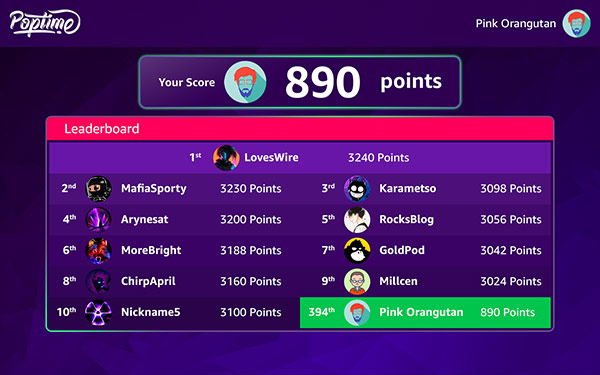Design and Implement Leaderboard for GamesFREE

Game Design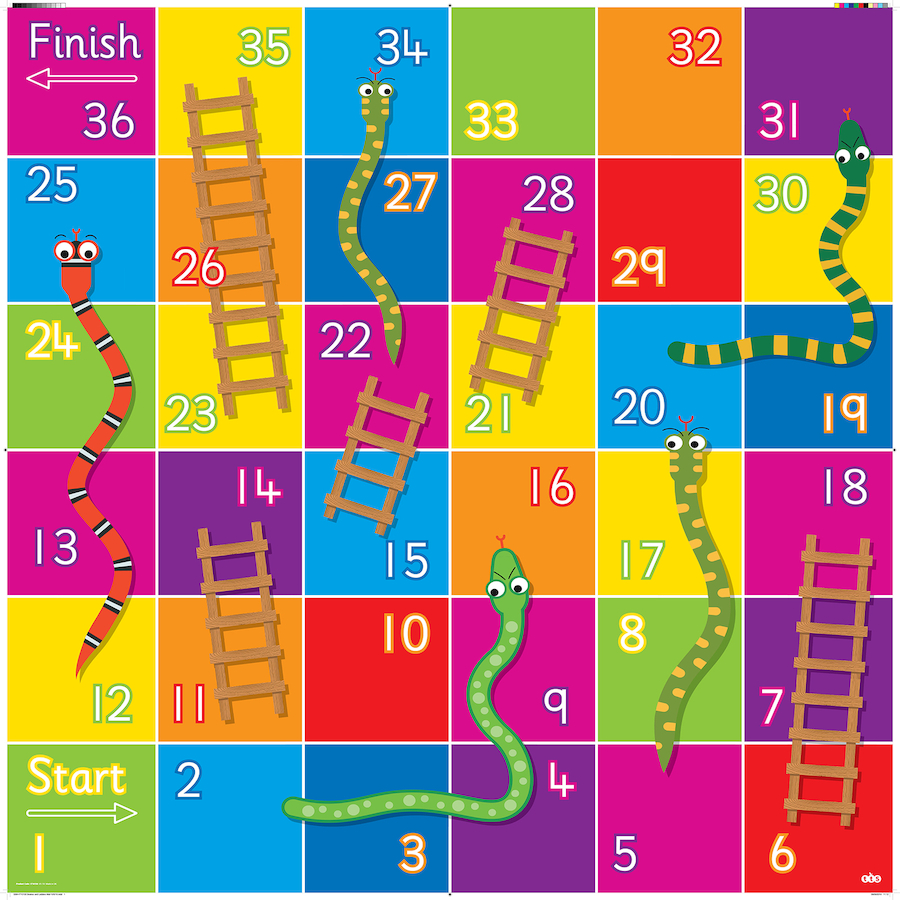Design and Implement Snake and Ladder GameFREE

Object Oriented DesignDesign BookMyShowFREE

Instructor:Abhishek Dey

Senior SDE | Chief Architect

Microsoft |
University of Florida

If you have any feedback, please use this form:
https://thealgorists.com/Feedback.```